#### Low-discrepancy sampling methods

Due to the fundamental importance of numerical integration and the intricate link between discrepancy and integration error, most sampling literature has led to low-discrepancy sequences and point sets [738,893,937]. Although motion planning is quite different from integration, it is worth evaluating these carefully constructed and well-analyzed samples. Their potential use in motion planning is no less reasonable than using pseudorandom sequences, which were also designed with a different intention in mind (satisfying statistical tests of randomness).

Low-discrepancy sampling methods can be divided into three categories: 1) Halton/Hammersley sampling; 2) (t,s)-sequences and (t,m,s)-nets; and 3) lattices. The first category represents one of the earliest methods, and is based on extending the van der Corput sequence. The Halton sequence is an-dimensional generalization of the van der Corput sequence, but instead of using binary representations, a different basis is used for each coordinate . The result is a reasonable deterministic replacement for random samples in many applications. The resulting discrepancy (and dispersion) is lower than that for random samples (with high probability). Figure 5.8a shows the firstHalton points in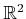.

Chooserelatively prime integers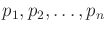(usually the firstprimes,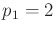,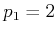,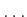, are chosen). To construct theth sample, consider the base-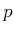representation for, which takes the form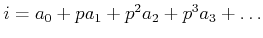. The following point in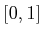is obtained by reversing the order of the bits and moving the decimal point (as was done in Figure 5.2):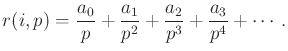(5.24)

For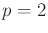, this yields theth point in the van der Corput sequence. Starting from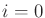, theth sample in the Halton sequence is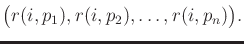(5.25)

Suppose instead that, the required number of points, is known. In this case, a better distribution of samples can be obtained. The Hammersley point set  is an adaptation of the Halton sequence. Using only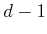distinct primes and starting at, theth sample in a Hammersley point set withelements is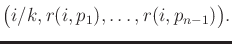(5.26)

Figure 5.8b shows the Hammersley set for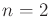and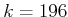.

The construction of Halton/Hammersley samples is simple and efficient, which has led to widespread application. They both achieve asymptotically optimal discrepancy; however, the constant in their asymptotic analysis increases more than exponentially with dimension . The constant for the dispersion also increases exponentially, which is much worse than for the methods of Section 5.2.3.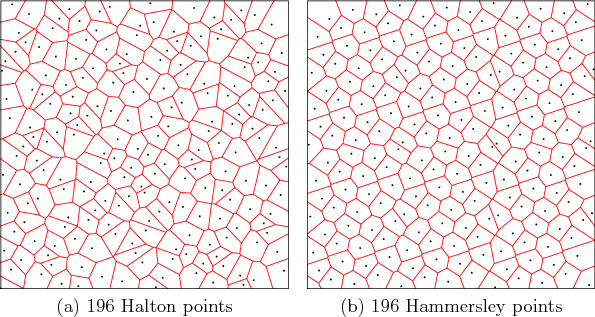Improved constants are obtained for sequences and finite points by using (t,s)-sequences, and (t,m,s)-nets, respectively . The key idea is to enforce zero discrepancy over particular subsets of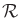known as canonical rectangles, and all remaining ranges inwill contribute small amounts to discrepancy. The most famous and widely used (t,s)-sequences are Sobol' and Faure (see ). The Niederreiter-Xing (t,s)-sequence has the best-known asymptotic constant,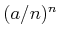, in which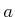is a small positive constant .

The third category is lattices, which can be considered as a generalization of grids that allows nonorthogonal axes [682,893,959]. As an example, consider Figure 5.5b, which showslattice points generated by the following technique. Let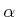be a positive irrational number. For a fixed, generate theth point according to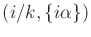, in which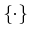denotes the fractional part of the real value (modulo-one arithmetic). In Figure 5.5b,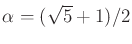, the golden ratio. This procedure can be generalized todimensions by picking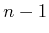distinct irrational numbers. A technique for choosing theparameters by using the roots of irreducible polynomials is discussed in . Theth sample in the lattice is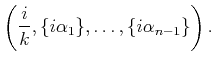(5.27)

Recent analysis shows that some lattice sets achieve asymptotic discrepancy that is very close to that of the best-known nonlattice sample sets [445,938]. Thus, restricting the points to lie on a lattice seems to entail little or no loss in performance, but has the added benefit of a regular neighborhood structure that is useful for path planning. Historically, lattices have required the specification ofin advance; however, there has been increasing interest in extensible lattices, which are infinite sequences [446,938].

Steven M LaValle 2020-08-14